## 2. Probabilities and distribution laws

 2.1 Binomial distribution (download) Plot the graphic of a Binomial distribution. Variables to customize: `prob <- 0.1# probabilityn <- 10 # number of independent experiments barthickness <- 20 # thickness of the graphical barscolour <- rgb (1,0,0,0.5) # colour of the bars and line`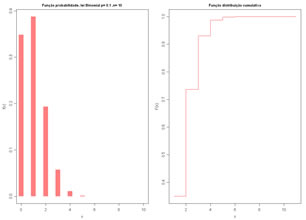2.2 Poisson distribution (download) Plot the graphic of a Poisson distribution. Variables to customize: `lambda <- 3 # lambda parameter (mean and variance)n <- 20 # number of independent experimentsndecimals <- 5 # number of decimal placesbarthickness <- 15 # thickness of the graphical barscolour <- rgb (1,0,0,0.5) # colour of the bars and line`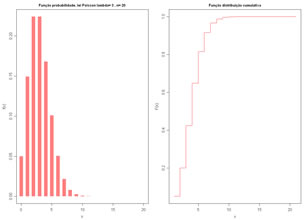2.3 Uniform distribution (download) Plot the graphic of an Uniform distribution and draw a set of values from the distribution. Variables to customize: `xmin <- 0.0 # minimum valuexmax <- 1000.0 # maximum valuendecimals <- 2 # number of decimalsnvalores <- 10 # number of values to generate by Monte Carlo simulationdiscretizacao <- 20 # number of points for graphical aproximationcolour <- rgb (1,0,0,0.5) # colour of the lines`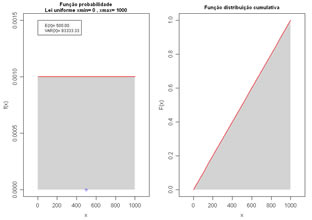2.4 Triangular distribution (download) Plot the graphic of a Triangular distribution and draw a set of values from the distribution. Variables to customize: `xmin <- 14.5 # minimum valuexmax <- 32.5 # maximum valuexmoda <- 30.0 # most likely valuendecimals <- 2 # number of decimalsnvalores <- 100 # number of values to generate by Monte Carlo simulationdiscretizacao <- 200 # number of points for graphical aproximationcolour <- rgb(1,0,0,0.5) # colour of the lines`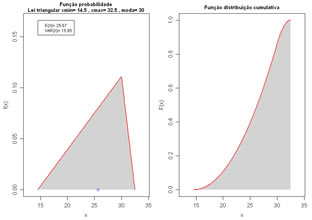2.5 Normal distribution (download) Plot the graphic of a Normal distribution and draw a set of values from the distribution. Variables to customize: `media <- 5.0 # mean of the normal distributiondesvp <- 2.0 # standard deviation of the normal distributionndecimals <- 2 # number of decimal placesnvalores <- 10000 # number of values to generate by Monte Carlo simulationdiscretizacao <- 200 # number of points for graphical aproximationcolour <- rgb (1,0,0,0.5) # colour of the lines`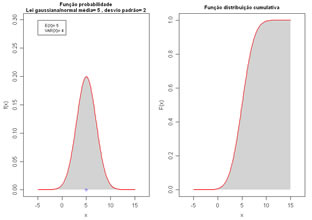2.6 Lognormal distribution (download) Plot the graphic of a Lognormal distribution and draw a set of values from the distribution. Variables to customize: `option <- 1 # can be (1) mean and standard deviation are of the normal distribution, or (2) mean and standard deviation are of the lognormal valuesmedia <- 5.0 # mean of the normal or lognormal distributiondesvp <- 2.0 # standard deviation of the normal or lognormal distributionndecimals <- 2 # number of decimal placesnvalores <- 10000 # number of values to generate by Monte Carlo simulationdiscretizacao <- 200 # number of points for graphical aproximationcolour <- rgb (1,0,0,0.5) # colour of the lines`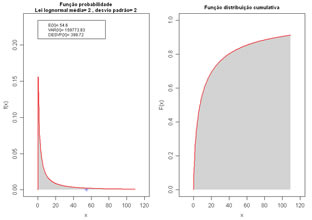2.7 Normal distributions comparison (download) Compare several Normal distributions, with different means and variances, in the same graph. Variables to customize: `media <- c(2,2,2,2,2) # mean of several normal distributions to comparedesvp <- c(1,2,3,4,5) # standard deviation of several normal distributions to comparediscretizacao <- 100 # number of points for graphical aproximationcolours <- c("red", "green", "blue", "yellow", "magenta")# list of colours of the several normal distributions to draw`2.8 Lognormal distribution comparison (download) Compare several Lognormal distributions, with different means and variances, in the same graph. Variables to customize: `media <- c(2,2,2,2,2) # mean of several lognormal distributions to comparedesvp <- c(1,2,3,4,5) # standard deviation of several lognormal distributions to comparediscretizacao <- 100 # number of points for graphical aproximationcolours <- c("red", "green", "blue", "yellow", "magenta") # list of colours of the several normal distributions to draw`2.9 Power distribution (download) Plot the graphic of a Power distribution. Variables to customize: `xmin <- 1 # minimum value of the distributionxmax <- 25 # maximum value of the distributionalfa <- 2 # power value of the distributionnclasses <- 25 # number of classes of the histogramdiscretizacao <- 1000 # number of points for graphical aproximationcolour <- rgb (1,0,0,0.5) # colour of the bars and lines`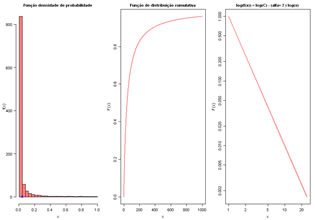2.10 Quantitative empirical distribution (download) Plot the empirical distribution of a quantitative variable (simple histogram and cumulative histogram) and add the normal or lognormal distribution curve with the same mean and variance of the data. Variables to customize: `varlistindex <- 5 # index of the GD frame quantitative variablevarunit <- "(ppm)" # units of the selected variablenclasses <- 15 # number of classes of the histogramfitmodel <- TRUE #fit a model (TRUE) or nor (FALSE)modeltype <- "lognormal" # type of model, normal or lognormal onlymaxY <- 1.0 # maximum value for Y axis in the left graphicdiscretizacao <- 40 # number of points for graphical aproximationcolourbar <- rgb(0,0,1,0.5) # colour of the histogram bars colourline <- rgb(1,0,0,0.5) # colour of the histogram lines`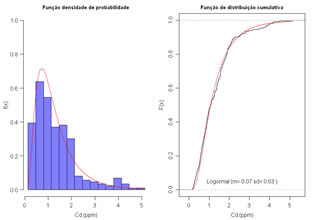2.11 Qualitative empirical distribution (download) Plot the empirical distribution of a qualitative variable (simple histogram and pseudo-cumulative histogram). Variables to customize: `varlistindex <- 4 # index of the GTD frame variablesortbyfreq <- TRUE # (TRUE) rank histogram by frequency in decreasing order or (FALSE) present histogram by alfabetic ordercolourbar <- rgb(1,0,0,0.5) # colour of the histogram barscolourline <- rgb(1,0,0,0.5)# colour of the histogram lines`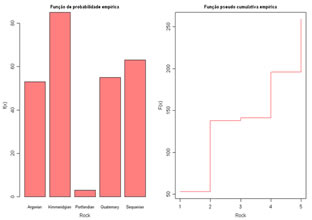2.12 Mix normal distributions (download) Use the Monte Carlo simulation to build a composite distribution mixing several normal distributions with different means and variances. Variables to customize: `nsims <- 500 # number of simulations (values to generate)probs <- c(0.25,0.25,0.5) # proportions of each normal distribution, values must sum one.media <- c(12,15,18) # means of several normal distributions to mixdesviop <- c(1,1,1) # standard variations of several normal distributions to mixnclasses <- 25 # number of classes in the mixure histogramvaloravalia <- 15 # evaluate the probability of being below this value colour <- rgb(0,0,1,0.5) # colour of the histogram bars and lines`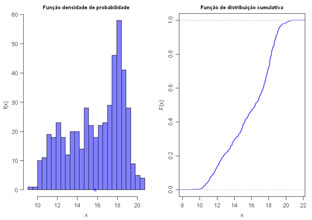2.13 Mix uniform distributions (download) Use the Monte Carlo simulation to build a composite distribution mixing several uniform distributions with different intervals (means and variances). The composite distribution is based on a simplified formula of Benefits B = PV x Q – CE x Q – CF Variables to customize: `nsims <- 500 # number of simulations (values to generate)Q <- 10000 # value for parameter minPV <- 4 # minimum value for PV variable uniformly distributedmaxPV <- 6 # maximum value for PV variable uniformly distributedminCE <- 1.5 # minimum value for CE variable uniformly distributedmaxCE <- 3.5 # maximum value for CE variable uniformly distributedminCF <- 13000 # minimum value for CF variable uniformly distributedmaxCF <- 15000 # maximum value for CF variable uniformly distributedvaloravalia <- 15 # evaluate the probability of being below this valueavalianclasses <- 10 # number of classes in the mixure histogramcolour <- rgb (0,0,1,0.5) # colour of the histogram bars and lines`